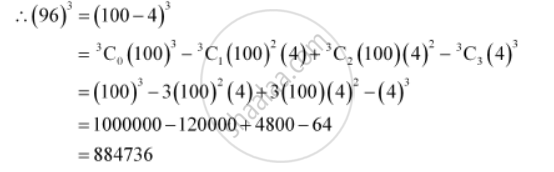CBSE (Arts) Class 11CBSE
Share

# Using Binomial Theorem, Evaluate (96)3 - CBSE (Arts) Class 11 - Mathematics

ConceptBinomial Theorem for Positive Integral Indices

#### Question

Using Binomial Theorem, evaluate (96)3

#### Solution

96 can be expressed as the sum or difference of two numbers whose powers are easier to calculate and then, binomial theorem can be applied.

It can be written that, 96 = 100 – 4Is there an error in this question or solution?

#### APPEARS IN

Solution Using Binomial Theorem, Evaluate (96)3 Concept: Binomial Theorem for Positive Integral Indices.
S